# Algebra II : Distributing Exponents (Power Rule)

## Example Questions

← Previous 1 3 4 5 6 7 8 9 12 13

### Example Question #1 : Distributing Exponents (Power Rule)

What is the largest positive integer,, such that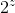is a factor of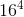?

Possible Answers:

5

8

10

20

16

Correct answer:

16

Explanation: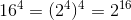. Thus,is equal to 16.

### Example Question #1 : Integer Exponents

Simplify the expression: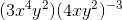Possible Answers: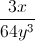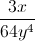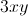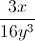Cannot be simplified

Correct answer:Explanation:

Begin by distributing the exponent through the parentheses. The power rule dictates that an exponent raised to another exponent means that the two exponents are multiplied: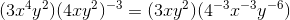Any negative exponents can be converted to positive exponents in the denominator of a fraction: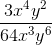The like terms can be simplified by subtracting the power of the denominator from the power of the numerator: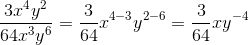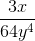### Example Question #2 : Distributing Exponents (Power Rule)

Order the following from least to greatest: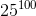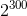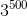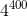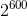Possible Answers: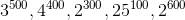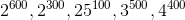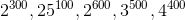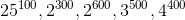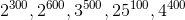Correct answer:Explanation:

In order to solve this problem, each of the answer choices needs to be simplified.

Instead of simplifying completely, make all terms into a form such that they have 100 as the exponent.  Then they can be easily compared.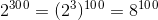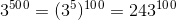,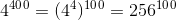, and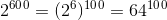.

Thus, ordering from least to greatest: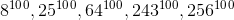.

### Example Question #3 : Distributing Exponents (Power Rule)

Simplify: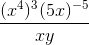Possible Answers: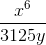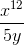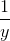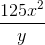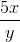Correct answer:Explanation:Step 1: Distribute the exponents in the numberator.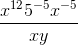Step 2: Represent the negative exponents in the demoninator.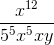Step 3: Simplify by combining terms.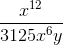### Example Question #2 : Integer Exponents

Simplify: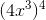Possible Answers: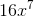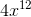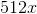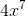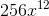Correct answer:Explanation:

Use the power rule to distribute the exponent: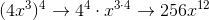### Example Question #4 : Distributing Exponents (Power Rule)

Simplify: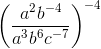Possible Answers: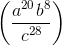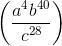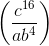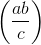Correct answer:Explanation:

Step 1: Distribute the exponent through the terms in parentheses: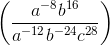Step 2: Use the division of exponents rule.  Subtract the exponents in the numerator from the exponents in the denominator: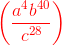### Example Question #5 : Distributing Exponents (Power Rule)

Simplify.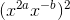Possible Answers: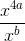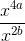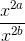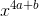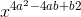Correct answer:Explanation:

When a power applies to an exponent, it acts as a multiplier, so 2a becomes 4a and -b becomes -2b. The negative exponent is moved to the denominator.

### Example Question #6 : Distributing Exponents (Power Rule)

Simplify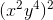.

Possible Answers: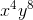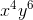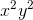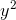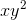Correct answer:Explanation:

When faced with a problem that has an exponent raised to another exponent, the powers are multiplied: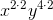then simplify:.

### Example Question #7 : Distributing Exponents (Power Rule)

Solve: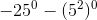Possible Answers: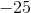Correct answer:Explanation:

Solve each term separately.  A number to the zeroth power is equal to 1, but be careful to apply the signs after the terms have been simplified.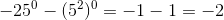### Example Question #8 : Distributing Exponents (Power Rule)

Simplify this expression: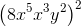Possible Answers: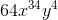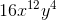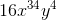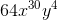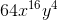Correct answer:Explanation:is the correct answer because the order of operations were followed and the multiplication and power rules of exponents were obeyed. These rules are as follows: PEMDAS (parentheses,exponents, multiplication, division, addition, subtraction), for multiplication of exponents follow the format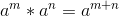, and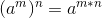.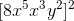First we simplify terms within the parenthesis because of the order of operations and the multiplication rule of exponents: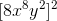Next we use the power rule to distribute the outer power: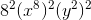=**note that in the first step it isn't necessary to combine the two x powers because the individuals terms will still add to x^16 at the end if you use the power rule correctly. However, following the order of operations is a great way to avoid simple math errors and is relevant in many problems.

← Previous 1 3 4 5 6 7 8 9 12 13

### All Algebra II Resources